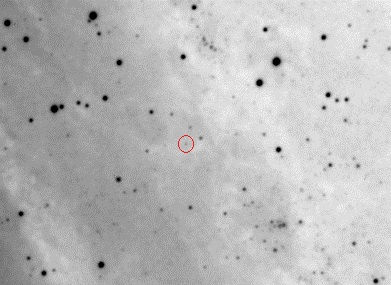top of page

## The star that changed the course of modern astronomy

###### Introduction

Among the millions of stars that we can observe with the instrumentation of an amateur astronomy, highlights a star that marked a before and after in the history of modern astronomy. At the beginning of the last century, most astronomers considered that everything we could observe, including spiral nebulae (that is how spiral galaxies were called), were part of our galaxy, the Milky Way. However, in 1927 the astronomer Edwin Hubble with the 2.50 meter telescope on Mount Wilson in California began to study the Andromeda galaxy, and discovered variable stars of the Cepheid type that were known in our galaxy and allowed to determine the distance based on the period of pulsation of its luminosity. Studying these stars in Andrómda, Hubble determined that Andromeda was 900,000 light years away, which placed it outside of our galaxy and meant a discovery of the first magnitude.

The first Cepheid variable that he discovered is the one called V1, and the challenge of the project explained below is to try to replicate the measurements that Hubble made with a 2.5m telescope by means of my 0.28m telescope. Hubble had the advantage of a large aperture, but he used photographic plates against the modern CCD technique. Still, it is a major challenge, given that the V1 star has a magnitude of 19.4 and that implies a very weak star to be measured with an 11-inch telescope installed in an urban environment.

###### Image capture and identification of V1

A photo taken with CCD is shown below, resulting from stacking 20 photos of 10 minutes. The field of view of the photo is 30x20 minute arcs centered on the coordinates of V1. The star V1 is marked with an arrow and is seen as a very weak star:A zoom is shown on the area of ​​the star V1 by inverting the previous photo:###### Photometric measurements

The purpose of the project was to measure the star V1 photometrically for two months, trying to take photos every 2 days. Since M31 V1 is a very faint star, between 18.5 and 19.4 of magnitude, with an 11-inch telescope it is difficult to work with filters that reduce the luminosity, so the photo were taken without a filter. The approach was to make 20 photos of 10 minutes each night. In each photo the signal-to-noise ratio of the star V1 is only 2 or 3, so it is necessary to perform the photometry measurement on the 20 photos stacked, thus achieving a signal-to-noise ratio in the star V1 between 10 and 15.

Finally, due to availability limitations and weather conditions, photos have been taken for 16 nights between 08/31/2016 and 10/15/2016. In total there are 320 photos of 10 minutes, more than 53 accumulated hours. Including the processing time, realization of the brightness curve, approximate period calculation, etc., is a project of more than 60 hours, but the result is worth it.

The equipment used is C11 telescope on mount EQ8, camera ST8XME without filter. The capture software is Maxim DL controlled by the ACP DC3 software.

For photometric measurements, the corresponding function of Maxim DL was used, selecting as the reference star the one indicated in the AAVSO star plotter shown below:The result of the photometric measurements is shown below:In the graph you can see an oscillation of the magnitude between 18.4 and 19.4. To calculate the period, a sinusoidal graph of the form A * sin (B * C + C1) + D has been represented. The light curve that can be found on the Internet from Hubble measurements shows that it is not exactly a sine wave, in fact the curve obtained shows how the rise is faster than the descent, but for an approximate calculation of the period, find a sinusoid approaching a complete cycle I consider to be valid

For the graphic representation of both the captured and the sinusoid points, the Graphical Analisys program has been used. The error values ​​have been calculated as the inverse of the signal-to-noise ratio.

Of the values ​​obtained in the adjustment of the sinusoid, what matters to us is B, with a value of 0.21. To obtain the period in days we use the following expression: Period = 2 * PI / B, obtaining a data of 29.9 days. Given that the accepted value is of the order of 31.4, a measurement of the period with a reasonable approximation has been achieved. Probably the error is due to the fact that the number of points represented is not enough and it would be necessary to cover more long time captures, instead of one and a half months, with daily takes.

Note: this method of calculating the period is what I used in my beginnings analyzing variables, now I prefer to use commercial analysis tools such as Peranso.

###### Calculation of the distance

For a Type I Cepheid like V1, the following relationship is currently considered valid:

Mv = -2.81 log (P) - 1.43

Using the calculated period of 29.9 we obtain:

Mv = -5.56

Mv is the absolute magnitude, and since we know the apparent magnitude (19.4) we can calculate the distance by applying the formula:

m-Mv = 5 * logD-5, where D is the distance in parsecs and m the apparent magnitude.

D = 10 ^ ((9.6 + 5.56) / 5 + 1) = 1028016 parsec, ie approximately 1 megaparsec. The data currently admitted is 0.89 megaparsec, so the Andromeda distance measurement has been achieved with a value with reasonable accuracy for an amateur team.In our case:

bottom of page